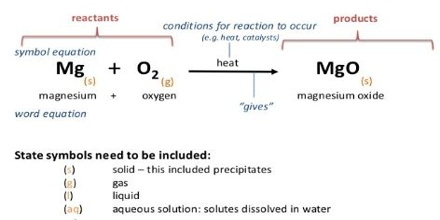# Conventions in Heat of Reaction

Conventions in Heat of Reaction

The Heat of Reaction (also known Enthalpy of Reaction) is the change in the enthalpy of chemical reaction that happens at a constant pressure.

(a) Changes of state an accompanied by evolution or absorption of the latent heat. The heat of reaction should depend on the states of the substances involved in the reaction. Thus in the formation of water;

H2(g) + ½ O2 (g) → H2O

If the H2O is in the liquid stale the heat of reaction will differ from that observed if the H2O is vapour. In writing appropriate equations in thermo-chemistry the state of the substance, i.e. whether it is gaseous, liquid, solid or in solution, is mentioned by writing the symbols (g), (l), (s) or (aq) respectively for the state after the chemical formula of the substance. Further, the heat of reaction depends on which crystalline from participated in the traction. Example in figure.For these reasons ∆H0 values are expressed with reference to reactions with the compounds in certain standard states. The states which by convention have been agreed upon by reference states in tabulating heats of reaction are summarized in Table.

Table: Standard States of SubstancesAgain the heat of reaction will depend on the pressure of the gases involved. The symbol ∆H indicates that the reaction takes place at constant pressure. The value at constant pressure is mentioned in most cases because the reactions are ordinarily carried out in open container under atmospheric pressure. If the reaction would have been carried out under constant volume conditions the heat of the reaction would be different in those cases involving gases where there is a difference in number of moles of reactants and products.

Heats of reaction have been given specials names in some cases depending on the type of the reaction. For example, reaction (a) shown in table above is a combustion reaction as carbon burns in oxygen to form carbon dioxide. The heat content change in this reaction is termed the heat of combustion (∆HC). This is also termed the heat of formation (∆HF) of carbon dioxide. There are heats of atomization, heats of dissociation, heats of precipitation, heats of neutralization, heats of hydrogenation etc. These are all heats of reaction.